Trigonometry in the Right Triangle and with the Unit Circle

Anderson Gomes Da Silva, Jeff Calareso
• Author
Anderson Gomes Da Silva

Anderson holds a Bachelor's and Master's Degrees (both in Mathematics) from the Fluminense Federal University and the Pontifical Catholic University of Rio de Janeiro, respectively. He was a Teaching Assistant at the University of Delaware (UD) for two and a half years, leading discussion and laboratory sessions of Calculus I, II and III. In the Winter of 2021 he was the sole instructor for one of the Calculus I sections at UD.

• Instructor
Jeff Calareso

Jeff teaches high school English, math and other subjects. He has a master's degree in writing and literature.

Learn about the unit circle in trigonometry. Understand the use of the unit circle to find the trigonometric functions. See how to find sine and cosine from a unit circle and how to find the tangent using unit circle sine-cosine ratios. Updated: 09/28/2021

Show

Trigonometric Relations in the Right Triangle

A right angle is an angle that measures {eq}90^{\circ} {/eq} (or {eq}\frac{\pi}{2} {/eq} if we want to give the measure in radians). Throughout this lesson, we are not going to stick to one unit to give the measure of an angle.

Now, a right triangle is a triangle with one of the interior angles measuring {eq}90^{\circ}, {/eq} like the triangle {eq}ABC {/eq} with sides {eq}a, b {/eq} and {eq}c {/eq} in the following figure.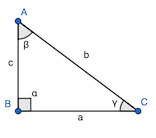The biggest side, opposed to the right angle, is called the hypothenuse, and the other two sides are the legs. In a right triangle, sine of an angle is defined as the ratio between the opposite side and the hypothenuse, cosine is the ratio between the adjacent side and the hypothenuse, and tangent is the ratio between the sine and cosine of the angle, or, equivalently, the ratio between the opposite side and the adjacent one. Defining the trigonometric ratios in the triangle {eq}ABC {/eq} for {eq}\beta {/eq} gives

$$\sin \beta = \frac{a}{b}, \cos \beta = \frac{c}{b} ~ \textrm{and} ~ \tan \beta = \frac{a}{c}$$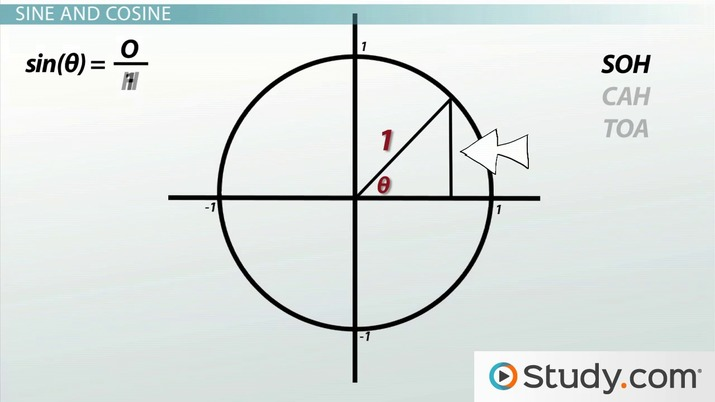An error occurred trying to load this video.

Try refreshing the page, or contact customer support.

Coming up next: Graphing Sine and Cosine

You're on a roll. Keep up the good work!

Replay
Your next lesson will play in 10 seconds
• 0:05 Everything Is Connected
• 0:54 Right Triangles and Trig
• 2:38 Sine and Cosine
• 3:33 Unit Circle Triangles
• 4:50 Lesson Summary
Save Save

Want to watch this again later?

Timeline
Autoplay
Autoplay
Speed Speed

Unit Circle Triangles

The idea of sine and cosine can be applied for every real number, and not only for the angles of a right triangle. The notion of a tangent, however, cannot be extended to some numbers because it is the ratio between the sine and the cosine. Because it is a fraction, the denominator cannot be zero. When we expand the trigonometric concepts, it is necessary to use a unit circle, which is simply a circle of radius {eq}1 {/eq} centered at the origin {eq}O(0, 0), {/eq} as shown in the image.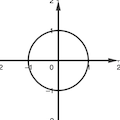The coordinate axes divide the Cartesian plane into four regions, called quadrants, and defined in the following list, which contains a fancy symbolic notation that will be explained afterward:

• First quadrant: {eq}Q_1 = \{(x, y) \in \mathbb{R}^2 | x > 0, y > 0\} {/eq}
• Second quadrant: {eq}Q_2 = \{(x, y) \in \mathbb{R}^2 | x < 0, y > 0\} {/eq}
• Third quadrant: {eq}Q_3 = \{(x, y) \in \mathbb{R}^2 | x < 0, y < 0\} {/eq}
• Fourth quadrant: {eq}Q_4 = \{(x, y) \in \mathbb{R}^2 | x > 0, y < 0\} {/eq}

The first quadrant is the set of points in the Cartesian plane where both the abscissa and the ordinate are positive. The explanation of the definition of the other quadrants is analogous and, for this reason, will be omitted. In the next image, we show the four quadrants and the coordinate axes.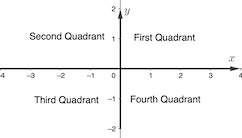Let's see a couple of unit circle triangles in a figure because they will help us when defining the trigonometric functions.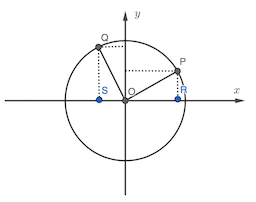Observe the triangles {eq}OPR {/eq} and {eq}OQS. {/eq} We are going to refer to right triangles formed by the origin, a point on the circumference, and points on the coordinate axes as unit circle triangles. They will be helpful when defining trigonometric functions. Rays {eq}\overrightarrow{OP} {/eq} and {eq}\overrightarrow{OR} {/eq} form two angles, as well as {eq}\overrightarrow{OR} {/eq} and {eq}\overrightarrow{OQ}. {/eq} In trigonometry, we adopt the convention that a counterclockwise rotation beginning on the positive x-axis gives a positive angle, whereas a clockwise one, a negative angle.

Trig Functions Using the Unit Circle

Now we are ready to see the definitions of the trig functions using the trig unit circle. Consider the last example where we have a right triangle {eq}OPR, {/eq} with {eq}P(x, y) {/eq} being a point on the circumference and {eq}\alpha {/eq} being the angle depicted below.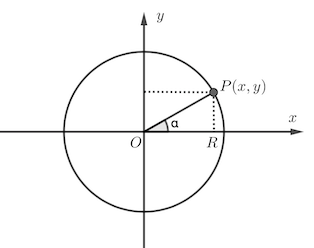Using the definition of sine, cosine, and tangent that we gave at the beginning for angles in the right triangle we get: {eq}\sin \alpha = \frac{y}{1}, \cos \alpha = \frac{x}{1} ~ \textrm{and} ~ \tan \alpha = \frac{y}{x} {/eq} In general, a point on the unit circle will determine an angle with the positive x-axis and the abscissa of such point will be the angle's cosine, the ordinate will be the angle's sine, and the ratio of the two will be the angle's tangent (provided that the cosine is not zero). In particular, for the angles {eq}0 {/eq} and {eq}\frac{\pi}{2} {/eq} the points on the unit circle will be {eq}(1, 0) {/eq} and {eq}(0, 1), {/eq} respectively. Thus, {eq}\sin 0 = 0, \sin \frac{\pi}{2} = 1, \cos 0 = 1 ~ \textrm{and} ~ \cos \frac{\pi}{2} = 0. {/eq}

To unlock this lesson you must be a Study.com Member.

What is the unit circle in trigonometry?

In trigonometry, the unit circle is a circle of radius 1 centered at the origin, that is, the point (0, 0)

What is cos on the unit circle?

Let O(0, 0) and P(x, y) be a point on the unit circle. The cosine of the angle between the positive x-axis and OP is x, the abscissa of P.

Register to view this lesson

Are you a student or a teacher?